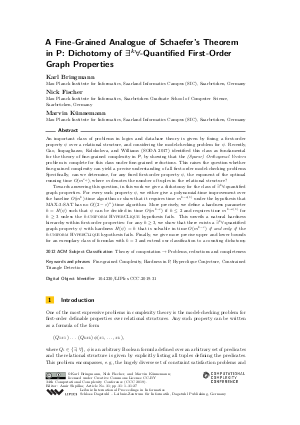Document# A Fine-Grained Analogue of Schaefer’s Theorem in P: Dichotomy of Exists^k-Forall-Quantified First-Order Graph Properties

### Authors Karl Bringmann, Nick Fischer, Marvin Künnemann## File

LIPIcs.CCC.2019.31.pdf
• Filesize: 0.67 MB
• 27 pages

## Cite As

Karl Bringmann, Nick Fischer, and Marvin Künnemann. A Fine-Grained Analogue of Schaefer’s Theorem in P: Dichotomy of Exists^k-Forall-Quantified First-Order Graph Properties. In 34th Computational Complexity Conference (CCC 2019). Leibniz International Proceedings in Informatics (LIPIcs), Volume 137, pp. 31:1-31:27, Schloss Dagstuhl - Leibniz-Zentrum für Informatik (2019)
https://doi.org/10.4230/LIPIcs.CCC.2019.31

## Abstract

An important class of problems in logics and database theory is given by fixing a first-order property psi over a relational structure, and considering the model-checking problem for psi. Recently, Gao, Impagliazzo, Kolokolova, and Williams (SODA 2017) identified this class as fundamental for the theory of fine-grained complexity in P, by showing that the (Sparse) Orthogonal Vectors problem is complete for this class under fine-grained reductions. This raises the question whether fine-grained complexity can yield a precise understanding of all first-order model-checking problems. Specifically, can we determine, for any fixed first-order property psi, the exponent of the optimal running time O(m^{c_psi}), where m denotes the number of tuples in the relational structure? Towards answering this question, in this work we give a dichotomy for the class of exists^k-forall-quantified graph properties. For every such property psi, we either give a polynomial-time improvement over the baseline O(m^k)-time algorithm or show that it requires time m^{k-o(1)} under the hypothesis that MAX-3-SAT has no O((2-epsilon)^n)-time algorithm. More precisely, we define a hardness parameter h = H(psi) such that psi can be decided in time O(m^{k-epsilon}) if h <=2 and requires time m^{k-o(1)} for h >= 3 unless the h-uniform HyperClique hypothesis fails. This unveils a natural hardness hierarchy within first-order properties: for any h >= 3, we show that there exists a exists^k-forall-quantified graph property psi with hardness H(psi)=h that is solvable in time O(m^{k-epsilon}) if and only if the h-uniform HyperClique hypothesis fails. Finally, we give more precise upper and lower bounds for an exemplary class of formulas with k=3 and extend our classification to a counting dichotomy.

## Subject Classification

##### ACM Subject Classification
• Theory of computation → Problems, reductions and completeness
##### Keywords
• Fine-grained Complexity
• Hardness in P
• Hyperclique Conjecture
• Constrained Triangle Detection

## Metrics

• Access Statistics
• Total Accesses (updated on a weekly basis)
0